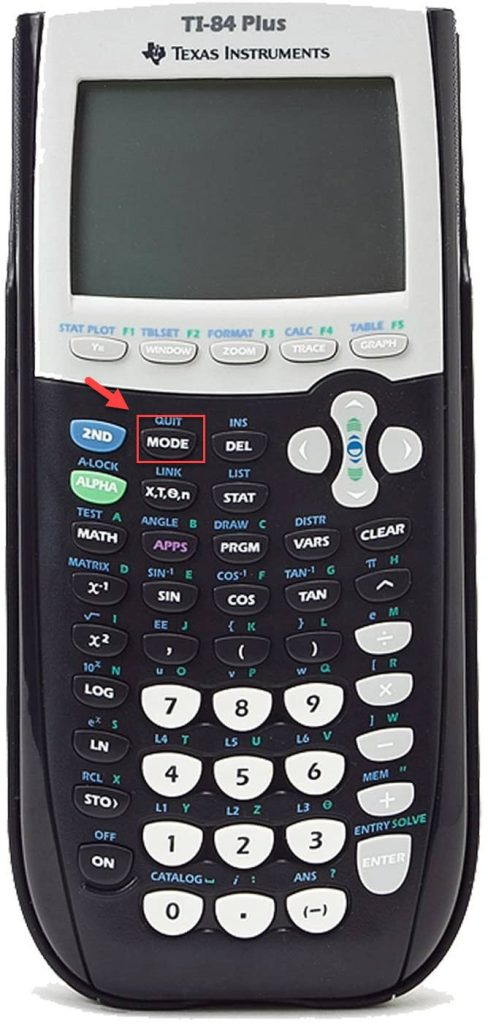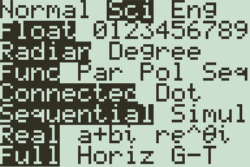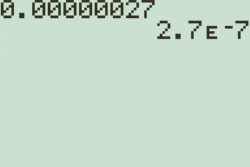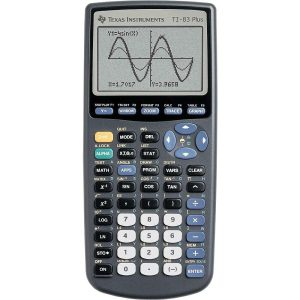# How To Do Scientific Notation On Calculator

Numbers can sometimes be too large or too small to be expressed normally. If you’re working on a problem involving the weight of the earth or the size of an atom, writing down or entering several zeroes in a calculator is inconvenient at best.

Writing scientific notation is one of the easiest ways of expressing extremely large and infinitesimally small numbers. For instance, you can express 0.0000000027 as 2.7 x 10-9

You can enter a scientific notation on scientific calculators. Every scientific calculator is also capable of outputting scientific notations per the rules of significant figures.

Here’s a short guide on how to do scientific notation on scientific calculators.

# How To Do Scientific Notation on Calculator

You can both enter and receive scientific notation as an output on your calculator.

## How to Convert a Number to Scientific Notation

To convert a number into a scientific notation or to set the calculator to output all the answers in that form, you have to change the mode of the calculator to the scientific mode.

You can do this in three easy steps.

### Step #1: Find the “Sci” Mode

Press the “MODE” button on your calculator. It is typically the second button on the top row of keys.Rows of options will appear on the calculator’s screen. The first row of options will include “Normal,” “Sci,” and “Eng” (Engineering notation mode).

Use the arrow keys on the calculator to navigate to the “Sci” option, then hit the “Enter” key.With this, you will have enabled the scientific notation converter mode.

### Step #2: Exit the Menu

To exit the MODE menu, tap the “2ND” button, and press the MODE button. You will return to the default screen of the calculator.

### Step #3: Calculate/Convert

Every calculation that you make on the calculator will now be displayed in scientific notation. If you want to convert a number into scientific notation, you must feed it in and hit enter. You will see that the scientific notation calculator will have moved the decimal places automatically.The “E” in the calculator means “exponent of 10.” In other words, “2.7 E -7” denotes “2.7 x 10-7”.

## How to Enter Scientific Notation in Calculators

### In a TI-30 Calculator

If you have a TI-30, and you want to enter 2.7 x 10-7 in your calculator. First, you must feed “2.7” into the calculator using the number keys.

Next, press the “2ND” key to activate the shift mode. Once activated, press the “x-1” key. Since the button has “EE” marked above it, it will feed the “E” symbol into the calculator.

You can now type in the exponent you want to enter, which in this case is “-7.”

### In a TI-83/84 CalculatorEntering scientific notation in these calculators is a little trickier. You must switch to the “Sci” mode to ensure that the scientific notation you enter isn’t converted to regular digits.

You can then enter the coefficient, press the 2ND key, then the comma key, since it has the “EE” marked above it. You can then enter the exponent, and you will have entered a scientific notation in your calculator.

We have various comprehensive calculators that you can use online for free. You can choose from t-test calculator, graphing, matrix, the standard deviation to statistics, and scientific calculators. Check it here